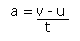## Acceleration

The “suvat” Equations

Acceleration is the rate of change of velocity of an object.where a is acceleration, v is the final velocity of the object, u is the initial velocity of the object and t is the time that has elapsed.

This equation can be rearranged to give:

• v = u + at

If s represents the displacement of the object, then:

• s = ½ (u + v)t

• s = ut + ½ at2

• v2 = u2 + 2as

These equations are true if the acceleration of the body in question is constant (i.e. it doesn’t change over the time period). The units used must be consistent, and the standard units are:

Acceleration: ms-2(or m/s2)
Velocity: ms-1 (or m/s)
Displacement: m
Time: s

The equation which you will need to use depends upon the question.

The Acceleration due to Gravity

If a body is dropped from a height, it will accelerate because of gravity. The acceleration caused by gravity is written as “g”, and is usually taken to be 9.8 ms-2.

Example

A ball is dropped from the leaning tower of Pisa, at a height of 50m from the ground. The ball is dropped from rest and falls freely under gravity. How long will it be before the ball hits the ground?

We know: s = 50, a = 9.8, u = 0 and we want to find t.

The equation connecting these four is s = ut + ½ at2

So 50 = 0 + ½ × 9.8 × t2
Rearranging: t2 = 10.20408
t = 3.19…
the time taken is 3.19s (3sf)